Note

This documentation is for a development version. Click here for the latest stable release (v1.3.0).

# Transitioning to nengo_spa coming from the core Nengo legacy SPA implementation¶

This tutorial is intended for persons who are already familiar with the legacy Semantic Pointer Architecture (SPA) implementation that was shipped with core Nengo until at least version 2.7. Thus, it will not explain any of the background behind the SPA and will reference concepts used in the legacy implementation. If you are completely new to the SPA, you might want to start with this tutorial that assumes no knowledge of the legacy SPA implementation.

## Why switch to nengo_spa?¶

You might wonder why you should switch to nengo_spa, if you have been using the legacy SPA and it was working well for you. Here is a number of reasons to prefer nengo_spa:

### Support for action rules of arbitrary complexity¶

A rule as complex as dot((role * filler + BiasVector) * tag, cmp) >> ..., which includes two circular convolutions and a dot product of two non-fixed values, is possible with nengo_spa. This was not possible with the legacy SPA implementation.

### “Type safety” in action rules¶

If different vocabularies are combined an explicit conversion is required. This prevents hard-to-track-down bugs. This conversion is also explicit about how the conversion is done, instead of just applying a fixed method that is not always appropriate.

### The neural representations optimized for better accuracy¶

That means less neurons are needed to achieve the same performance. This can make simulations run faster. This improvement of accuracy is comparable to the results presented in “Optimizing Semantic Pointer Representations for Symbol-Like Processing in Spiking Neural Networks” which achieved improvements of up to 25 times. In contrast to that paper, the implementation used in nengo_spa is purely based on setting appropriate radii and distributions for evaluation points as well as intercepts. This implementation is much faster.

### New Python-based syntax¶

A new Python-based syntax gives syntax highlighting and can make use of static checking tools like pylint to catch misspelled names and similar errors early.

### Support for algebras¶

The SPA uses circular convolution for binding by default, but other binding operations are also viable. Different algebras can be implemented with nengo_spa. In particular, an implementation of the vector-derived transformation binding (VTB) is provided, which is especially suited for deep structures.

### Other features¶

• The neural representation has been optimized to allow the representation of the identity vector (with the option to turn this optimization off).

• SPA networks can be used as and within normal Nengo networks. For example, instead of a basic and an SPA associative memory network, there is only one type of network that can be used in either case.

• SPA networks can be nested.

• Support for the Nengo config system.

• Scalars can now be routed and used as inputs/outputs using spa.Scalar.

• Lots of fixed issues and in general less possibilities to do things wrong.

## Importing nengo_spa¶

To save typing it is recommended to import nengo_spa as spa. Note that this is only a very small difference to import nengo.spa as spa which imports the legacy SPA. In the following, it is assumed that you imported nengo_spa as spa.

:

%matplotlib inline

import nengo
import numpy as np
import matplotlib.pyplot as plt

import nengo_spa as spa
from nengo_spa.examine import pairs
from nengo_spa.exceptions import SpaParseError, SpaTypeError

rng = np.random.RandomState(1)  # change this to change vocabularies

:

d = 32  # Default dimensionality to use in the examples


## Rule 1 of using nengo_spa¶

When using nengo_spa, use spa.Network() in any place where you would use a nengo.Network(). There isn’t any downside to using spa.Network() and in this way you will not run into problems of using nengo.Network() where spa.Network() is required. That being said, if you have existing nengo.Networks (that do not use any SPA features), these can stay nengo.Networks. In other words, you will only have to touch code using the SPA to upgrade to nengo_spa; networks not using the SPA system may stay as they are.

If you want to know when exactly spa.Network() is required, here are the conditions:

1. If you build action rules, they have to be build in a spa.Network().

2. Each network referenced in an action rule has to be a spa.Network().

## A basic model¶

:

with spa.Network(seed=0) as model:
state = spa.State(d)
bind = spa.Bind(d)

spa.sym.A * spa.sym.B >> state
state >> bind.input_left
~spa.sym.B >> bind.input_right

p = nengo.Probe(bind.output, synapse=0.03)


This defines a basic SPA model. It is conceptually similar to what you were to write in legacy SPA, but the syntax has changed considerably and there are some other fine differences.

The first difference is that instead of with spa.SPA() ..., we are using with spa.Network() .... There is no difference between the top-level spa.SPA and spa.Module anymore. Everything is just a spa.Network.

The next two lines are almost the same as for the legacy SPA. You define the modules with the vocabulary dimension as before. There is, however, no need anymore to assign them as attributes to model. But you are free to do so, if you prefer.

The next lines are what previously was implemented with nengo.spa.Cortical. Now these rules can be written down directly in Python code without a special object. Each >> means, take the left side and route it to the input an the right side. (Previously this was done with the assignment operator = and the other way around.) When accessing specific inputs of a module, standard dot notation is used (instead of an underscore). Also note that the names of some of the inputs have changed. They follow a consistent naming scheme now: always starting with input_ and you use the same name in action rules as in manually created Nengo connections. Because everything is stated in pure Python code now, you have to be explicit about when you are using a symbolic reference to a semantic pointer. That is done with the spa.sym. prefix. The vocabulary from which the specified semantic pointer is taken will be inferred from the context.

:

with nengo.Simulator(model) as sim:
sim.run(1.0)


Let us plot the result. Note that we can access the d-dimensional default vocabulary with model.vocabs[d].

:

plt.plot(sim.trange(), spa.similarity(sim.data[p], model.vocabs[d]))
plt.xlabel("Time [s]")
plt.ylabel("Similarity")
plt.legend(model.vocabs[d].keys(), loc="best")

:

<matplotlib.legend.Legend at 0x7f2c9f1dfe50>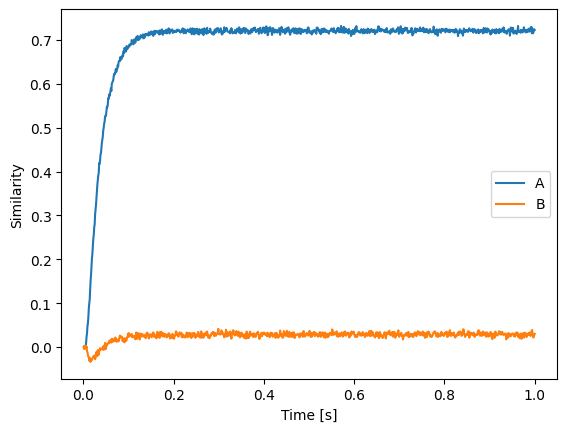There is another detail you might notice here: the keys attribute on the vocabularies is now a function. This is to have Vocabulary adhere to the usual Python API of dictionaries and mapping types.

## Transcode¶

In the previous example, input was provided directly in the action rules. But this does not allow for time-varying inputs. The legacy SPA Input has been replaced with spa.Transcode which has an API more similar to classic nengo.Nodes. It takes either a constant value or a function which may return any of the following:

• A string that is parsed as a semantic pointer.

• A symbolic Semantic Pointer expression.

• A SemanticPointer instance.

• A NumPy array.

The next examples demonstrates this. It also manually specifies a vocabulary, but ignore this for now as the changes to Vocabulay will be discussed later.

:

vocab = spa.Vocabulary(d, strict=False, pointer_gen=rng)

def stimulus_fn(t):
if t < 0.5:
return "A * B"  # Return string to be parsed
elif t < 1.0:
return vocab.parse("C * B")  # Return SemanticPointer instance
else:
return np.zeros(d)  # Return a numpy array

with spa.Network(seed=0) as model:
state = spa.State(vocab)
bind = spa.Bind(vocab)
spa_input = spa.Transcode(stimulus_fn, output_vocab=vocab)

spa_input >> state
state >> bind.input_left
~spa.sym.B >> bind.input_right

p = nengo.Probe(bind.output, synapse=0.03)

:

with nengo.Simulator(model) as sim:
sim.run(1.5)

:

plt.plot(sim.trange(), spa.similarity(sim.data[p], vocab))
plt.xlabel("Time [s]")
plt.ylabel("Similarity")
plt.legend(vocab.keys(), loc="best")

:

<matplotlib.legend.Legend at 0x7f2ca1925790>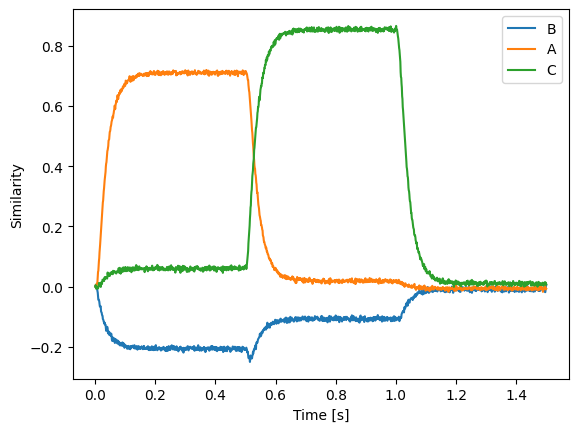In addition to providing output, you can now use the spa.Transcode module to provide an input from the network. Its output function gets three inputs: the current simulation time, a SemanticPointer instance of the input, and the input vocabulary. To create a Transcode instance, the dimensionality (or vocabulary) has to be passed in twice. The first specifies the input vocabulary (the one passed to the output function), the second one specifies the output vocabulary that will be used to parse the function’s output.

In the following example we compute the circular convolution with ~B in math instead of a neural network.

:

def cconv(t, pointer):
return pointer.vocab.parse("~B") * pointer

with spa.Network(seed=0) as model:
state = spa.State(d)
bind = spa.Transcode(cconv, d, d)
spa_input = spa.Transcode("A * B", output_vocab=d)

spa_input >> state
state >> bind

p = nengo.Probe(bind.output, synapse=0.03)

:

with nengo.Simulator(model) as sim:
sim.run(1.0)

:

plt.plot(sim.trange(), spa.similarity(sim.data[p], model.vocabs[d]))
plt.xlabel("Time [s]")
plt.ylabel("Similarity")
plt.legend(model.vocabs[d].keys(), loc="best")

:

<matplotlib.legend.Legend at 0x7f2c9ee9cbb0>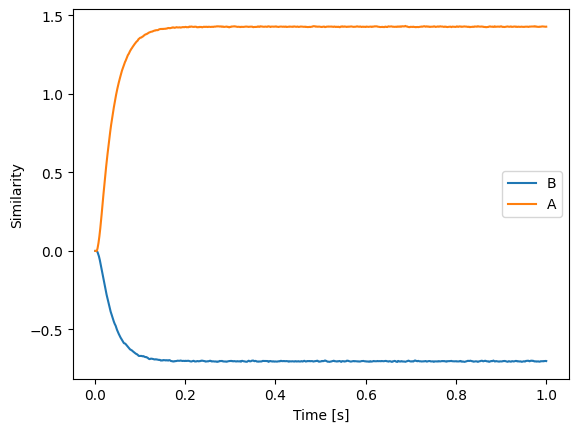## Action rules¶

We have already seen how to create cortical action rules with nengo_spa. The next example shows how to add action rules implemented through the basal ganglia-thalamus loop. It is the classical routing through a sequence example.

The action rules have to be defined in the context of a spa.ActionSelection object (you are allowed to have multiple independent ActionSelection objects). Each rule is defined with the spa.ifmax function. The first argument is an expression that provides the utility value, and all remaining arguments are routings to do when that rules utility value is the largest.

:

def start(t):
if t < 0.05:
return "A"
else:
return "0"

with spa.Network(seed=0) as model:
state = spa.State(d)
spa_input = spa.Transcode(start, output_vocab=d)

spa_input >> state
with spa.ActionSelection():
spa.ifmax(spa.dot(state, spa.sym.A), spa.sym.B >> state)
spa.ifmax(spa.dot(state, spa.sym.B), spa.sym.C >> state)
spa.ifmax(spa.dot(state, spa.sym.C), spa.sym.D >> state)
spa.ifmax(spa.dot(state, spa.sym.D), spa.sym.E >> state)
spa.ifmax(spa.dot(state, spa.sym.E), spa.sym.A >> state)

p = nengo.Probe(state.output, synapse=0.01)

:

with nengo.Simulator(model) as sim:
sim.run(0.5)

:

plt.plot(sim.trange(), spa.similarity(sim.data[p], model.vocabs[d]))
plt.xlabel("Time [s]")
plt.ylabel("Similarity")

:

Text(0, 0.5, 'Similarity')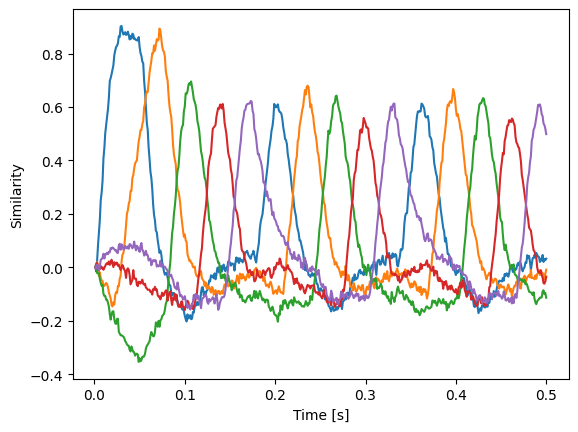There is no need to manually create the basal ganglia and thalamus anymore. This happens automatically. You can access these objects as the bg and thalamus attributes of the ActionSelection object. This example also demonstrates how to name actions by using a optional first (!) argument to ifmax.

:

def start2(t):
if t < 0.05:
return "A"
else:
return "0"

with spa.Network(seed=0) as model:
state = spa.State(d)
spa_input = spa.Transcode(start2, output_vocab=d)

spa_input >> state
with spa.ActionSelection() as action_sel:
spa.ifmax("state == A", spa.dot(state, spa.sym.A), spa.sym.B >> state)
spa.ifmax("state == B", spa.dot(state, spa.sym.B), spa.sym.C >> state)
spa.ifmax("state == C", spa.dot(state, spa.sym.C), spa.sym.D >> state)
spa.ifmax("state == D", spa.dot(state, spa.sym.D), spa.sym.E >> state)
spa.ifmax("state == E", spa.dot(state, spa.sym.E), spa.sym.A >> state)

p_thalamus = nengo.Probe(action_sel.thalamus.output, synapse=0.01)
p_utility = nengo.Probe(action_sel.bg.input, synapse=0.01)

:

with nengo.Simulator(model) as sim:
sim.run(0.5)

:

plt.subplot(2, 1, 1)
plt.plot(sim.trange(), sim.data[p_thalamus])
plt.legend(action_sel.keys(), fontsize="x-small")
plt.ylabel("Thalamus output")

plt.subplot(2, 1, 2)
plt.plot(sim.trange(), sim.data[p_utility])
plt.legend(action_sel.keys(), fontsize="x-small")
plt.ylabel("Utility")

:

Text(0, 0.5, 'Utility')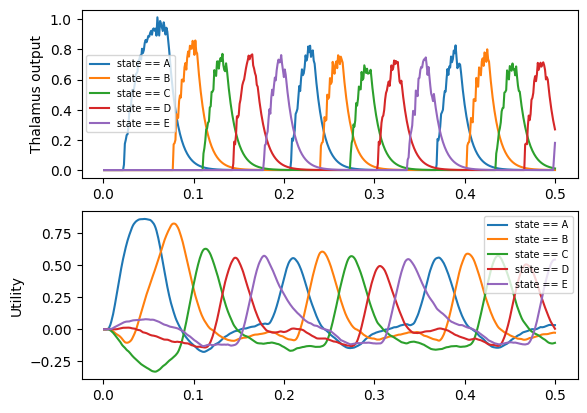Opposed to the legacy SPA, nengo_spa allows arbitrarily complex actions rules. In the following example we define dot products between two states and dynamic circular convolutions. All required networks will be created automatically.

:

with spa.Network(seed=0) as model:
state_ab = spa.State(d)
state_a = spa.State(d)
state_b = spa.State(d)
out = spa.State(d)

spa.sym.A * spa.sym.B >> state_ab
spa.sym.A >> state_a
state_ab * ~state_a >> state_b

with spa.ActionSelection():
spa.ifmax(spa.dot(state_ab, state_a * state_b), state_ab * ~spa.sym.B >> out)
spa.ifmax(0.5, spa.sym.C >> out)

p = nengo.Probe(out.output, synapse=0.01)

:

with nengo.Simulator(model) as sim:
sim.run(0.5)

:

plt.plot(sim.trange(), spa.similarity(sim.data[p], model.vocabs[d]))
plt.xlabel("Time [s]")
plt.ylabel("Similarity")
plt.legend(model.vocabs[d].keys())

:

<matplotlib.legend.Legend at 0x7f2c9ebb9df0>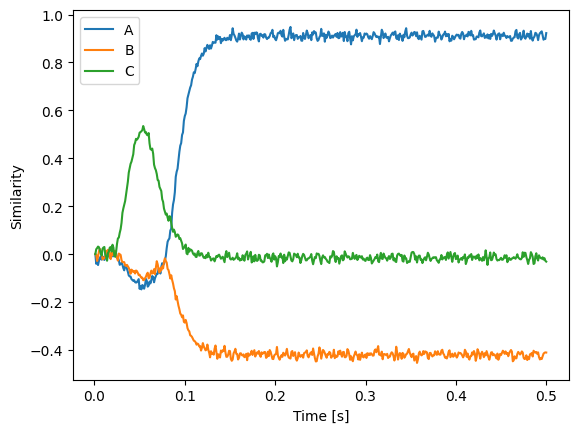Note that there is no automatic sharing of networks in the action rules. If, for example, you define the same circular convolution in there, two such networks will be created. But you can define a single circular convolution, assign it to a variable, and reuse that variable to reuse the circular convolution network like so:

cconv = state1 * state2
cconv * ~POINTER1 >> state3
cconv * ~POINTER2 >> state4


If you have nengo_gui installed, it can be used to take a look at all the things that get created.

:

try:
from nengo_gui.ipython import IPythonViz

vis = IPythonViz(model)
except (ImportError, AttributeError):
print("GUI not installed or code not executed in Jupyter notebook.")
vis = None
vis

GUI not installed or code not executed in Jupyter notebook.


## Vocabularies¶

Nengo_spa behaves a lot like legacy SPA when providing dimensionalities only. Vocabularies will implicitly be created according to the specified dimensions and Semantic Pointers will be automatically added. When creating a vocabulary explicitly, things are a little bit different. In that case the vocabulary will be in strict-mode by default. That means an exception will be raised when trying to parse a Semantic Pointer that is not in the vocabulary. This is to prevent accidentally adding new Semantic Pointers (something that tended to happen with the associative memory in legacy SPA) and can make it easier to notice typing errors.

:

vocab = spa.Vocabulary(d, pointer_gen=rng)
try:
vocab.parse("A")
except SpaParseError as err:
print(err)

Error parsing expression 'A' with 32-dimensional vocab  at 0x7f2c9886b4f0: name 'A' is not defined


All Semantic Pointers have to be added to the vocabulary with either add or the new populate method before they are recognized by parse. You can add multiple pointers add once by separating them with a semicolon in populate.

:

vocab = spa.Vocabulary(d, pointer_gen=rng)
vocab.populate("B; C")
vocab.parse("A + B + C")

:

SemanticPointer(array([ 3.05703289e-02,  5.17268764e-01, -3.05163733e-02, -1.65742905e-01,
4.07998650e-04, -4.66466802e-01,  1.85889223e-01, -9.84536190e-02,
2.05388329e-01,  6.66899980e-01, -1.31254620e-01, -3.40330950e-02,
2.41410023e-01, -5.17499157e-01, -2.38508011e-01,  2.88028693e-01,
2.79172931e-02,  4.23138182e-01, -1.77870802e-01, -5.20428358e-01,
-3.18948002e-01,  2.50932866e-01,  2.45376879e-01,  2.72511956e-01,
3.70793384e-01, -4.11998873e-01,  5.07650926e-01,  3.00410895e-01,
-3.06841313e-01,  3.36849637e-01, -6.91286211e-02, -2.82017544e-01]), vocab=<nengo_spa.vocabulary.Vocabulary object at 0x7f2c935d8ee0>, algebra=<nengo_spa.algebras.hrr_algebra.HrrAlgebra object at 0x7f2ca19d6790>, name='A + B + C'


If you prefer to automatically add unknown vectors, you can disable strict mode. This can be especially useful when experimenting with initial ideas in the GUI.

:

vocab = spa.Vocabulary(d, strict=False, pointer_gen=rng)
vocab.parse("A")

:

SemanticPointer(array([-0.02289058, -0.13421333,  0.04758596, -0.13906621,  0.10455379,
0.16095464, -0.11882202,  0.20077817, -0.04005542,  0.19448251,
0.07376143,  0.18893406, -0.16783948, -0.01797782,  0.2443353 ,
-0.10404104, -0.34294483, -0.32701773, -0.42193715, -0.35590082,
-0.06929582,  0.21522891, -0.07444539,  0.05446297, -0.0185161 ,
0.00143914, -0.02831479, -0.02932172,  0.0776329 , -0.1979663 ,
0.17004599,  0.15487689]), vocab=<nengo_spa.vocabulary.Vocabulary object at 0x7f2c935e7460>, algebra=<nengo_spa.algebras.hrr_algebra.HrrAlgebra object at 0x7f2ca19d6790>, name='A'


The new populate method is much more powerful than adding pointers with parse. For example you can use existing Semantic pointers to construct new ones and you can use transforms as normalized() and unitary() to make vectors unit length or normalized. Note that a simple Semantic Pointer will be normalized, but you need to explicitly do this when constructing a pointer out of others.

In the following example we create a vocabulary with four pointers A, B, C, and D. A is made unitary, D is constructed from other vectors and normalized.

:

vocab = spa.Vocabulary(d)
vocab.populate("A.unitary(); B; C; D = (A * B + C).normalized()")


Another new and sometimes useful method is parse_n which allows to parse multiple Semantic Pointer expressions at once. This can be useful for programmatically constructing a list of pointers for plotting. The following example demonstrates that for all convolution pairs. It also shows that when using a predefined vocabulary, the modules will obtain their dimensionality from that vocabulary. No need to pass dimensionality and vocabulary anymore!

:

vocab = spa.Vocabulary(d, pointer_gen=rng)
vocab.populate("A; B; C")

with spa.Network() as model:
state = spa.State(vocab)
spa.sym.A * spa.sym.B >> state
p = nengo.Probe(state.output, synapse=0.01)

:

with nengo.Simulator(model) as sim:
sim.run(0.5)

:

plot_keys = pairs(vocab)
plot_vectors = vocab.parse_n(*plot_keys)

plt.plot(sim.trange(), spa.similarity(sim.data[p], plot_vectors))
plt.xlabel("Time [s]")
plt.ylabel("Similarity")
plt.legend(plot_keys)

:

<matplotlib.legend.Legend at 0x7f2c93896940>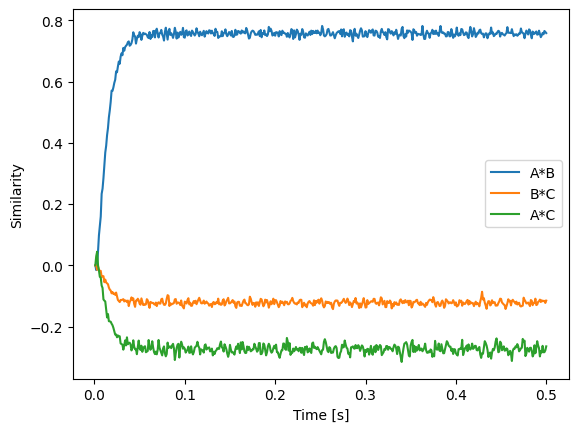Another feature of nengo_spa is “type-safety” in the sense that you cannot just connect things with different vocabularies in action rules.

:

v1 = spa.Vocabulary(d)
v1.populate("A; B")
v2 = spa.Vocabulary(d)
v2.populate("B; C")

try:
with spa.Network() as model:
state1 = spa.State(v1)
state2 = spa.State(v2)
state2 >> state1
except SpaTypeError as err:
print(err)

Different vocabularies: TVocabulary<32-dimensional vocab  at 0x7f2c93896640>, TVocabulary<32-dimensional vocab  at 0x7f2c93899130>


If we want to do this, we have to be explicit about how the conversion between the vocabularies is supposed to be happen. The first option (if both vocabularies have the same dimensionality) is to just reinterpret the Semantic Pointer in the other vocabulary. Because the vectors in both vocabularies are independent (by default) the A from v2 will be different from A in v1.

:

v1 = spa.Vocabulary(d, pointer_gen=rng)
v1.populate("A; B")
v2 = spa.Vocabulary(d, pointer_gen=rng)
v2.populate("A; B")

with spa.Network() as model:
state1 = spa.State(v1)
state2 = spa.State(v2)

spa.reinterpret(state2) >> state1
spa.sym.A >> state2

p = nengo.Probe(state1.output, synapse=0.01)

:

with nengo.Simulator(model) as sim:
sim.run(0.5)

:

plt.plot(sim.trange(), v1["A"].dot(sim.data[p].T))
plt.plot(sim.trange(), v2["A"].dot(sim.data[p].T))
plt.xlabel("Time [s]")
plt.ylabel("Similarity")
plt.legend(["v1['A']", "v2['A']"])

:

<matplotlib.legend.Legend at 0x7f2c9e934460>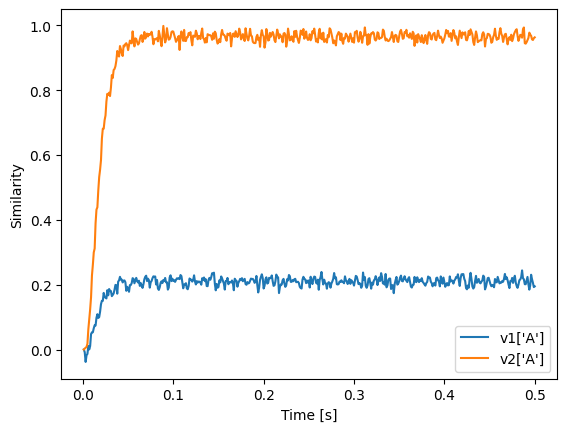We see that the value in state2 is still similar to v2’s A even though state2 uses v1 as vocabulary.

The second choice to convert between vocabularies is translate which will construct a transformation matrix to convert from one vocabulary to the other based on the Semantic Pointer names. This also works with vocabularies that do not match in dimensionality, but the target vocabulary should contain all keys of the source vocabulary. If this is not the case, you will get either a warning or an exception depending on whether you are using strict-mode vocabularies. You can also use the populate=True argument to translate to have all missing keys added to the target vocabulary.

:

v1 = spa.Vocabulary(d, pointer_gen=rng)
v1.populate("A; B")
v2 = spa.Vocabulary(d, pointer_gen=rng)
v2.populate("A; B")

with spa.Network() as model:
state1 = spa.State(v1)
state2 = spa.State(v2)

spa.translate(state2, v1) >> state1
spa.sym.A >> state2

p = nengo.Probe(state1.output, synapse=0.01)

:

with nengo.Simulator(model) as sim:
sim.run(0.5)

:

plt.plot(sim.trange(), v1["A"].dot(sim.data[p].T))
plt.plot(sim.trange(), v2["A"].dot(sim.data[p].T))
plt.xlabel("Time [s]")
plt.ylabel("Similarity")
plt.legend(["v1['A']", "v2['A']"])

:

<matplotlib.legend.Legend at 0x7f2c9eb2f0a0>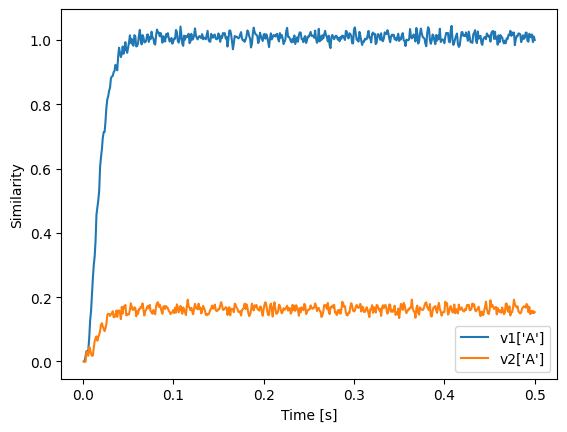## nengo_spa and the config system¶

One of the major improvements in nengo_spa is the extensive use of Nengo’s config system. For example it allows to set the vocab for all states globally.

:

with spa.Network() as model:
model.config[spa.State].vocab = d

state1 = spa.State()
state2 = spa.State()

state1 >> state2
spa.sym.A >> state1

p = nengo.Probe(state2.output, synapse=0.01)

:

with nengo.Simulator(model) as sim:
sim.run(0.5)

:

plt.plot(sim.trange(), spa.similarity(sim.data[p], model.vocabs[d]))
plt.xlabel("Time [s]")
plt.ylabel("Similarity")
plt.legend(model.vocabs[d].keys())

:

<matplotlib.legend.Legend at 0x7f2c9eaf5fa0>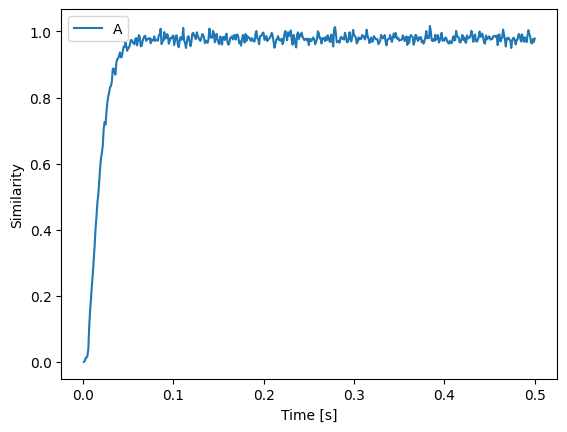The config system can also be used to set neuron numbers, subdimensions, and various other parameters, in particular of objects created when building action rules.

:

def start3(t):
if t < 0.1:
return "A"
else:
return "0"

with spa.Network(seed=0) as model:
model.config[spa.State].neurons_per_dimension = 10
model.config[spa.State].subdimensions = 1
model.config[spa.Thalamus].synapse_channel = nengo.Lowpass(0.1)

state = spa.State(d)
spa_input = spa.Transcode(start3, output_vocab=d)

spa_input >> state
with spa.ActionSelection():
spa.ifmax(spa.dot(state, spa.sym.A), 2 * spa.sym.B >> state)
spa.ifmax(spa.dot(state, spa.sym.B), 2 * spa.sym.C >> state)
spa.ifmax(spa.dot(state, spa.sym.C), 2 * spa.sym.A >> state)

p = nengo.Probe(state.output, synapse=0.01)

:

with nengo.Simulator(model) as sim:
sim.run(0.5)

:

plt.plot(sim.trange(), spa.similarity(sim.data[p], model.vocabs[d]))
plt.xlabel("Time [s]")
plt.ylabel("Similarity")
plt.legend(model.vocabs[d].keys())

:

<matplotlib.legend.Legend at 0x7f2c9f2a6580>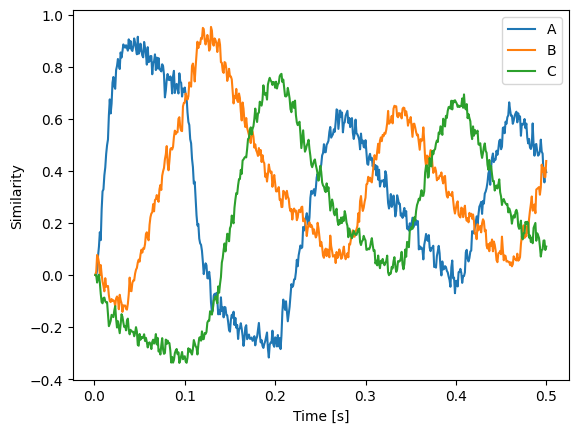## Semantic Pointers¶

The SemanticPointer class has become immutable in nengo_spa to avoid accidental modifiction of Semantic Pointers. Whenever an operation is applied to a SemanticPointer (for example SemanticPointer(d) + SemanticPointer(d) or SemanticPointer(d).normalized() a new instance will be created.

To facilitate mathematical analysis of encoding schemes some special vectors are predefined:

• nengo_spa.pointer.Identity(d) gives the identity vector for circular convolution.

• nengo_spa.pointer.Zero(d) gives the vector of all zeros (absorbing element for circular convolution).

• nengo_spa.pointer.AbsorbingElement(d) gives a vector that destroys all information under circular convolution except for a DC offset.

## Representing identity¶

In nengo_spa spa.State() is optimized to allow the representation of the identity vector. This is not always necessary as in many models the identity never needs to represented. With represent_identity=False this optimization can be disabled. This can make the representation for non-identity vectors slightly better. It also simplifies the internal structure of spa.State() which can be helpful for applying learning rules.

## New associative memory classes¶

To reduce the number of arguments of the associative memory class it has been split into two classes. spa.ThresholdingAssocMem is the applying a simple threshold, spa.WTAAssocMem performs a winner-take-all clean-up with lateral inhibitory connections. There is also a new type of associative memory spa.IAAssocMem based on independent accumulators, that also exhibits winner-take-all behaviour, but with different dynamics. For more information see the paper “A Spiking Independent Accumulator Model for Winner-Take-All Computation”.

To implement auto-associative memories, the input_keys and output_keys arguments have been replaced by a single mapping arguments.